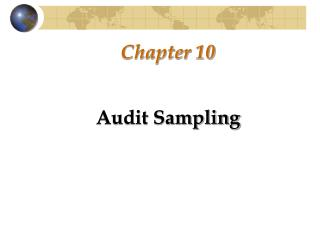DownloadDownload PresentationChapter 10

# Chapter 10

Télécharger la présentation## Chapter 10

- - - - - - - - - - - - - - - - - - - - - - - - - - - E N D - - - - - - - - - - - - - - - - - - - - - - - - - - -
##### Presentation Transcript

1. Chapter 10 Audit Sampling

2. Introduction Overview of Audit Sampling Definition Population Nonsampling and Sampling Risk Assessing control risk to high Assessing control risk to low

3. Sampling Risk: Substantive Testing • Risk of incorrect acceptance • Risk of incorrect rejection • Selecting a Sampling Approach • Statistical sampling • Nonstatistical sampling • Sampling to Test Controls • Attribute estimation sampling

4. Substantive Sampling Considerations • Basic Steps: • Specify audit objective • Define a misstatement • Define the population • Choose sampling method • Determine sample size • Selectthe sample • Audit the selected items • Evaluatethe sample results • Perform follow-up work as necessary • Document

5. Specify the Audit Objective • Combination of sampling and other audit procedures. • Sampling used in two ways: • Determine reasonableness of an account balance. • Estimate some amount.

6. Define Misstatements • Misstatements should be defined before beginning to: • preclude rationalizing away misstatements • provide guidance to the audit team • Misstatement: a difference that affects the correctness of the overall account balance.

7. Define the Population • Group of items in an account balance that the auditor wants to test. • Does not include: • items that the auditor has decided to examine 100%. • items that will be tested separately.

8. Define the Sampling Unit • Sampling units are the individual auditable elements as defined by the auditor. • Completeness of the Population • Auditor needs assurance that the list accurately represents the population.

9. Identify Individually Significant Items • Top stratum--few large-dollar items • Lower stratum--the remaining items • Stratification of the population

10. Choose a Sampling Method • Nonstatistical • Probability proportional to size (PPS) • Classical sampling methods (Appendix 10B) • Mean-per-unit • Ratio estimation • Difference estimation

11. Determine the Sample Size, Select the Sample, and Evaluate the Results • Tolerable misstatement • Expected misstatement • Document the Results

12. Nonstatistical Sampling • Determine sample size • Selecting the Sample • Haphazard selection • Evaluating the sample results • Using audit software

13. Probability Proportional to Size Sampling • PPS sampling is an adaptation of attribute sampling methods to dollar value testing. • Population definition • Test of details risk (incorrect acceptance) • Other substantive procedures risk (OSPR) • Sample size and selection • Zero and Negative Balances

14. PPS • Sample Evaluation • Error Evaluation Terminology • Upper misstatement limit (UML) • Basic precision • Most likely misstatement (MLM) • Incremental allowance for sampling error • Allowance for sampling error

15. Appendix 10A: Sampling to Test Controls • Planning Factors • Audit objective • Tolerable failure rate • Expected failure rate • Allowance for sampling error (precision) • Allowable risk of assessing control risk too low

16. Sampling to Test Controls • Nonstatistical sampling • Attribute Estimation sampling • Define the attributes of interest and failures • Define the population • Period covered by the tests • Sampling unit • Ensuring the population is complete

17. Sampling to Test Controls • Determine the Sample Size • Multiple attributes • Effect of population size (finite adj. factor) • Determine the method of selecting the sample • Random numbers • Systematic selection • Haphazard selection

18. Sampling to Test Controls • Select and audit the sample items. • Evaluate the sample results. • Quantitative evaluation • Qualitative evaluation • Linkage to substantive testing

19. Appendix 10B: Classical Sampling Methods • MPU Sampling • Difference estimation sampling • Ratio estimation sampling • Sample Size • Estimation of population standard deviation • Desired allowance for sampling error

20. Classical Sampling Methods • Sample Selection • Sample Evaluation • Point estimate • Projected misstatement • Achieved precision • Decision rules • Adjusted achieved precision • Unacceptable results

21. Classical Sampling Methods • Comparison of PPS and classical sampling • Computer support